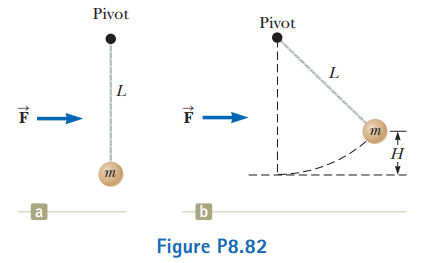# Problem: A ball of mass m = 300 g is connected by a strong string of length L = 80.0 cm to a pivot and held in place with the string vertical. A wind exerts constant force F to the right on the ball as shown in Figure P8.82. The ball is released from rest. The wind makes it swing up to attain maximum height H above its starting point before it swings down again.(a) Find H as a function of F. Evaluate H for(b) F = 1.00 N and(c) F = 10.0 N. How does H behave(d) as F approaches zero and(e) as F approaches infinity?(f) Now consider the equilibrium height of the ball with the wind blowing. Determine it as a function of F. Evaluate the equilibrium height for(g) F = 10 N and(h) F going to infinity.

###### FREE Expert Solution

This is a work-energy problem. The wind is doing work on the ball.

We'll need a suitable diagram(s) and work-energy equations to explain how F and H are related.

96% (121 ratings)###### Problem Details

A ball of mass m = 300 g is connected by a strong string of length L = 80.0 cm to a pivot and held in place with the string vertical. A wind exerts constant force F to the right on the ball as shown in Figure P8.82. The ball is released from rest. The wind makes it swing up to attain maximum height H above its starting point before it swings down again.

(a) Find H as a function of F. Evaluate H for
(b) F = 1.00 N and
(c) F = 10.0 N. How does H behave
(d) as F approaches zero and
(e) as F approaches infinity?
(f) Now consider the equilibrium height of the ball with the wind blowing. Determine it as a function of F. Evaluate the equilibrium height for
(g) F = 10 N and
(h) F going to infinity.Frequently Asked Questions

What scientific concept do you need to know in order to solve this problem?

Our tutors have indicated that to solve this problem you will need to apply the Motion Along Curved Paths concept. You can view video lessons to learn Motion Along Curved Paths. Or if you need more Motion Along Curved Paths practice, you can also practice Motion Along Curved Paths practice problems.

What professor is this problem relevant for?

Based on our data, we think this problem is relevant for Professor Schulte's class at UCF.

What textbook is this problem found in?

Our data indicates that this problem or a close variation was asked in Physics for Scientists and Engineers - Serway Calc 9th Edition. You can also practice Physics for Scientists and Engineers - Serway Calc 9th Edition practice problems.